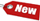Browse Accounting Lessons HereAccounting Terms & DefinitionsAccounting for Merchandising ActivitiesDebits and Credits (Double Entry Accounting)Business Valuation FormulasTime Value of Money & Present/Future ValuesComplex Debt & Equity InstrumentsCommon Stock & Shareholder's EquityAccounting & Finance RatiosValuing Common StockCorporate Income TaxesLower of Cost or Market (LCM) & Inventory ValuationChart of Accounts & BookkeepingBonds Payable & Long Term LiabilitiesCapital AssetsGAAP, Accrual & Cash Accounting, Information Commodity, Internal Controls & MaterialityWhat category of browser are you on this website? Accounting student (homework help) Finance professor (university research) Accounting manager (at work) Other Explore Careers in Accounting and FinanceVisit our section on Careers in Accounting & Finance to explore vast opportunities in this industry.

Chapter 4.7® - Present Value & Discounting – We Know the Future Value, how do we find the Present Value? Single Period Case & PV Discounting for Multiple Periods

When we discuss future value, we think of questions like “What will my \$5,000 grow to if it earns 7% interest every year for the next 10 years?” The answer to this question is to find the future value of this \$5,000 in 10 years, earning 7% return every year. The answer to this question is:

 N = 10 I/Y = 7% PV = \$-5,000 PMT = \$0 FV = ? 2ND I/Y P/Y = 1 C/Y = 1 CPT & FV = \$9,835.76

A similar type of question we could ask is, suppose we need to have \$20,000 in 10 years, earning only 6.5% interest pear year, how much do we need to invest today to reach our goal? This is called present value discounting and we elaborate more on this topic below..

Single Period Case

From the calculations we did in the Future Value & compounding interest section, we know that the future value of \$1 invested for one year at 10% is \$1.10. We now modify this question slightly and ask the following: How much do we have to invest today at 10% to get \$1 in one year? In other words, our desired future value is \$1, what is the present value (PV)? The answer to this question is quite simple actually. Whatever we invest today will be 1.1 times bigger at the end of the year (due to the 10% interest). Since we need \$1 at the end of the year:

 Present Value x 1.1 = \$1 Present Value = \$1/1.1 = \$0.909 Present Value = \$0.909 (90.9 cents)

The definition of this answer above is that, what amount invested today will grow to \$1 in one year if the interest rate is 10%? Present value is thus the reverse of future value and instead of compounding the money in to the future; we discount it backwards to the present!

Real Life example of Single Period Present Value Discounting

Suppose you need \$600 to buy textbooks for next academic year. You can earn 5% interest on your money, how much do you need to have today in order to save up that \$600 for next year?

In other words, we need to know the PV of \$600 in one year at 5% interest. Here are the calculations:

 Present value x 1.05 = \$600 Present Value = \$600 / 1.05 Present Value = \$571.43

Notice in our examples above, we assume we need to grow our money 1 year in to the future. What if we need to grow our money 2 or 3 or more years in to the future? This is called present value discounting for multiple periods, and we discuss this topic below.

Present Value Discounting for Multiple Periods

Suppose you need to have \$10,000 in two years. If you can earn 6% interest, how much do you have to invest today to make sure you have \$10,000 in two years, when you will need it? In other words, what is the present value of \$10,000 in two years if the relevant rate is 6%? Based on the previous discussion on future values, we know that the amount invested must grow to \$10,000 over 2 years. In other words, here’s how we can derive this in a formula:

 \$10,000 = PV x 1.062 \$10,000 = PV x 1.1236 PV = \$10,000 / 1.1236 PV = \$8,899.96